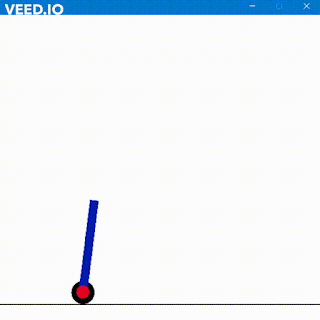## RING COUNTER

Hola Amigos
Today I will be discussing with you about Ring Counter.

A ring Counter is basically a simple counterthat counts upto n states working on the basics of shift registers. Upon each clock cycle the binary number shifts by 1 bit. Thus for n- bit binary number we have n-states.
Remember Johnsson counter works similar was but has n/2 states.

Heres a bsic diagram explaining Ring Counter

Let the input be 1000
Thus  we the following output

Here is the code for ring counter.
Give it a shot!!

Code
module D(d,reset,clk,q);
input d;
input clk;
input reset;
output q;
reg q;
initial q = 1'b1;
always @(posedge clk)
begin
if(reset==0)
q <= d;
else
q <= 1'b0;
end
endmodule

module D2(d,clk,q);
input d;
input clk;
output q;
reg q;
initial
q = 1'b0;
always @(posedge clk)
q <= d;
endmodule

module Ring_B();
reg clk;
reg reset;
wire [3:0]q;
initial
begin
reset = 1;
clk = 0;
#45 reset = 0;
end

D ini(q,reset,clk,q);  // for first FF
D2 rest1(q,clk,q);      //***
D2 rest2(q,clk,q);          // * These are rest FFs
D2 rest3(q,clk,q);      //***
always
#5 clk = !clk ;
endmodule

and here is the output

I will be coming soon with Twisted Ring Counter

So Long